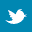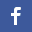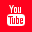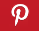Completely Solved C, C++ Programs Assignment.Quick Search Database Videos Tutorials Ebooks Forums FAQ Aboutus Household Industrial Manufacturing Service Shopping Transportation### C++ program to find the occurrences of the largest digit of a number within a set of numbers.

 Filed Under: C++ Assignments

Problem Statement :
Write a C++ program to find the occurrences of the largest digit of a number within a set of numbers.

Logical Approach :
The above program is done using an Abstract Data Type (ADT) called class. A class is a mechanism to create objects. Also we use constructor in this program to dynamically create and initialize array and destructor to de allocate memory space which was previously created. In this program a class named frequency is created.

It contains the following data members :
• size.
• Position.
• *arr. It contains the following member functions :
• input_no()- It is used to take input numbers of the array.
• get_pos()- It returns the position of the number user want to check.
• freq_cal()- It calculates and prints the maximum digit of the number and number of occurrences of that digit.
• freqency()- It is default constructor which is used to initialize the array.
• ~freqency()- It is destructor function which deallocates memory.

The program takes an array of numbers as input(the array is dynamically allocated in the default constructor and all the elements are set as 0 initially). Then it accepts any position of the array within the range from the user. Now it checks whether that element exists or not, if yes then it prints the frequency of the largest digit within that number and also finds the occurrence locations.
Example :

 12345 67589 92394 16547 23854

Given index=>3
The number is : 92394
The largest digit is : 9
The occurrence of the largest digit is : 2
The position(s) of the largest digit is : 1, 4.

Algorithm :
Creating an ADT with name frequency

Step 2:Declaring private data members int size;//Declaring an integer int position;//Declaring an integer int*arr;// Declaring an integer array whose memory space will be dynamically allocated

Step 3:Declaring public member functions input_no(); get_pos(); freq_cal(); freqency();//Default constructor ~freqency();//destructor

Default constructor is used to initialize the array

freqency()
{
Dynamically allocating number of instances of arr;
for(i=1 to size)//All the elements are initially 0
arr[i]=0;
}

Destructor function deallocates memory
~freqency()
{
delete arr;//Deallocating the allocated memory space
}

This function is used to take input numbers of the array
input_no()
{
for(i=1 to size)
{
}
}

This function returns the position of the number user want to check
get_pos()
{
while(True)
{
if(position>=1 AND position<=size)
Exit from loop;
Print ”Error!!Invalid position entered.";
}
return arr[position-1];
}

This function calculates and prints the maximum digit of the number and number of occurrences of that digit
freq_cal()
{
max=-99999;
t=num;
c=0;
while(num!=0)
{
tmp[i]=num%10;//Extracting digits of the number and store in reverse order in tmp[] array
if(tmp[i]>max)
max=tmp[i];
i=i+1;
num=num/10;
c=c+1;
}
co=0;//This counter counts number of occurrences of max digit Print max;//Print maximum digit
for(i=1 to c)
{
if(tmp[i]==max)
co=co+1;
}
for(i=c to 0)
{
if(tmp[i]==max)
Print c-i;//Print positions of max digit
}
}

Main procedure :
main()
{
freqency ob;
ob.input_no();
do
{
int x=ob.get_pos();
ob.freq_cal(x);
Print"Do you want to check for another position(Y/N):";
}while(choice=='Y'||choice=='y');
}

Program listing :

//C++ program to find the occurrences of the largest digit of a number within a set of numbers #include<iostream>
using namespace std;
class freqency//Creating a class freqency
{
private:
//Data members
int size;
int position;
int*arr ;
public:
//Member functions
void input_no();
int get_pos();
void freq_cal(int);
freqency();//Default constructor
~freqency();//destructor
};
//Default constructor is used to initialize the array
freqency::freqency()
{
cout<<"Enter size of the array:";
cin>>size;
arr=new int[size];//Dynamically allocating number of instances
for(int i=0;i<size;i++)//All the elements are initially 0
arr[i]=0;
}
//destructor function deallocates memory
freqency::~freqency()
{
delete[] arr;//Deallocating the allocated memory space
}
//This function is used to take input numbers of the array
void freqency::input_no()
{
for(int i=0;i<size;i++)
{
cout<<"\nEnter number
"<<i+1<<":";
cin>>arr[i];
}
}
//This function returns the position of the number user want to check
int freqency::get_pos()
{
while(1)
{
cout<<"\nEnter position of the number you want to
check:";
cin>>position;
if(position>=1&&position<=size)
break;
cout<<"\nError!!Invalid position entered.\n";
}
return arr[position-1];
}
//This function calculates and prints the maximum digit of the number and number of occurrences of that digit
void freqency::freq_cal(int num)
{
int tmp,i=0,max=-99999;
cout<<"\nThe number is:"<<num<<"\n";
int t=num;
int c=0;
while(num)
{
tmp[i]=num%10;//Extracting digits of the number and store in reverse order in tmp[] array
if(tmp[i]>max)
max=tmp[i];
i++;
num=num/10;
c++;
}
int co=0;//This counter counts number of occurrences of max digit
for(i=0;i<c;i++)
{
if(tmp[i]==max)
co++;
}
cout<<"Max digit "<<max<<" occurs
"<<co<<" time(s) in "<<t<<" and occurring position(s) are: ";
for(i=c-1;i>=0;i--)
{
if(tmp[i]==max)
cout<<c-i<<",";
}
cout<<"\b.";
}
int main()
{
freqency ob;
char ch;
ob.input_no();
do
{
int x=ob.get_pos();
ob.freq_cal(x);
cout<<"\n\nDo you want to check for another
position(Y/N):";
cin>>ch;
}while(ch=='Y'||ch=='y');
return 0;
}

Output :

Enter size of the array:5
Enter number 1:34553
Enter number 2:89969
Enter number 3:10895
Enter number 4:36678
Enter number 5:81776

Enter position of the number you want to check:2
The number is:89969

Max digit 9 occurs 3 time(s) in 89969 and occurring position(s) are: 2,3,5.

Do you want to check for another position(Y/N):Y

Enter position of the number you want to check:1
The number is:34553

Max digit 5 occurs 2 time(s) in 34553 and occurring position(s) are: 3,4.

Do you want to check for another position(Y/N):Y

Enter position of the number you want to check:7

Error!!Invalid position entered.

Enter position of the number you want to check:5
The number is:81776

Max digit 8 occurs 1 time(s) in 81776 and occurring position(s) are: 1.

Do you want to check for another position(Y/N):N

Discussions :

•   Namespaces allow to group entities like classes, objects and functions under a name. This way the global scope can be divided in "sub-scopes", each one with its own name.
•   All the files in the C++ standard library declare all of its entities within the std namespace. That is why we have generally included the using namespace std; statement in all programs that used any entity defined in iostream.
•  Error checking for the position of the number and size of array is included in the program. If the position entered is not in the range then an error messege is displayed. Similarly if size is less than or equal to zero an error messege is displayed.
•  C++ provides a special member function called the constructor whose task is to initialize the objects of its class. It is special because its name is same as the class name. C++ also provides another member function called the destructor that destroys the objects when they are no longer required. We have used constructor in this program to dynamically create and initialize array and destructor to de allocate memory space which was previously created.
•  We have used‘new’for dynamically creating array of instances.It is done in default constructor. The syntax is:*variableName= new[SIZE]
•  We should deallocate the memory space(by using delete) which was allocated previously for creating array of instances(After it is no longer required).It is done in destructor The syntax is: delete[]variableNameBack to main directory:Software Practical